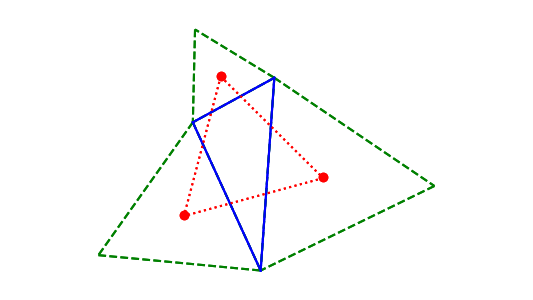# Napoleon’s theorem

The following theorem is attributed to Napoleon Bonaparte (1769–1821). There’s some debate over whether Napoleon was the first to discover the theorem, but I don’t believe there’s any doubt that the theorem, like Morley’s theorem from the previous post, was discovered a long time after Euclid.

Start with any triangle and draw equilateral triangles on each side. Then connect the centroids of the added triangles. The resulting triangle will be equilateral.In the diagram above, we start with the triangle with solid blue lines, then add the equilateral triangles with dashed green lines. The centroids of the green triangles are marked with red dots. The red triangle is equilateral.

See the next post for another version of Napoleon’s theorem.

## 2 thoughts on “Napoleon’s theorem”

1. Daniel Lemire

Does it generalize to rectangles ?

2. Euclydes Trovato

Bonaparte was highly profficient in geometry and applied systematically to military operations.
He authored a book on kinematic geometry principles, with deduc tion procedures!